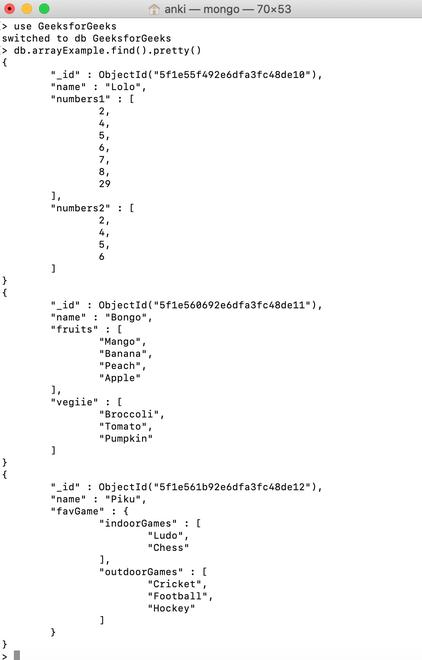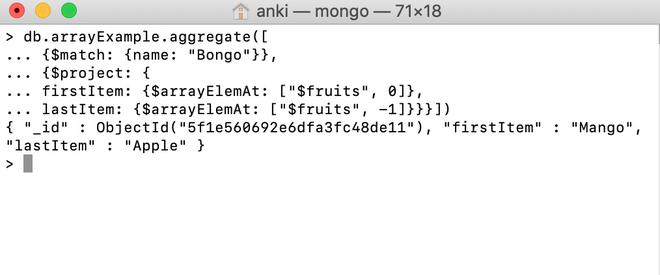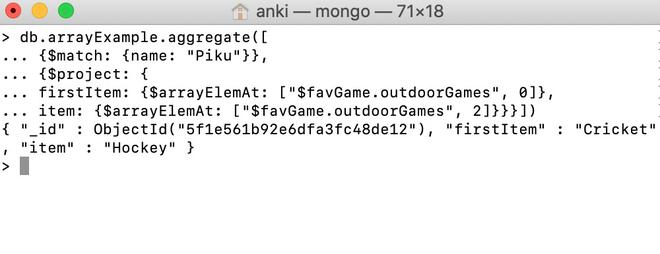GFG App
Open AppBrowser
Continue

MongoDB provides different types of array expression operators that are used in the aggregation pipeline stages and \$arrayElemAt operator is one of them. This operator is used to return the element present on the specified index of the given array.

Syntax:

`{ \$arrayElemAt: [ <array>, <index> ] }`

Here, the array must be a valid expression until it resolves to an array and index must be a valid expression until it resolves to an integer.

• If the value of index is positive, then this operator will return the element at the index position, from the start of the array.
• If the value of index is negative, then this operator will return the element at the index position, from the end of the array.
• If the index exceeds the array bounds, then this operator does not return any result.

Examples:

In the following examples, we are working with:

Database: GeeksforGeeks

Collection: arrayExample

Document: three documents that contain the details in the form of field-value pairs.Using \$arrayElemAt Operator:

In this example, we are going to find the elements of the array(i.e., the value of fruits field) on the specified index using \$arrayElemAt operator.

```db.arrayExample.aggregate([
... {\$match: {name: "Bongo"}},
... {\$project: {
... firstItem: {\$arrayElemAt: ["\$fruits", 0]},
... lastItem: {\$arrayElemAt: ["\$fruits", -1]}}}])```Using \$arrayElemAt Operator in the Embedded Document:

In this example, we are going to find the elements of the array(i.e., the value of favGame.outdoorGames field) on the specified index using \$arrayElemAt operator.

```db.arrayExample.aggregate([
... {\$match: {name: "Piku"}},
... {\$project: {
... firstItem: {\$arrayElemAt: ["\$favGame.outdoorGames", 0]},
... item: {\$arrayElemAt: ["\$favGame.outdoorGames", 2]}}}])```My Personal Notes arrow_drop_up